# Gravity Calculations - Neptune

Welcome! This site allows you to perform various gravity calculations based on Isaac Newton's law of universal gravitation.

NeptuneMass (kg)1.0243E+26
Mean Radius (km)24624
m (mass relative to Earth)17.147
r (mean radius relative to Earth)3.865
g-force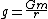1.148
Acceleration (m/s/s)11.257

#### Gravity Solutions

How far has an object fallen after t seconds? Equation: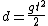[Latex: d=\frac{gt^2}{2}]

How fast is an object going after falling for t seconds? Equation: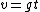[Latex: v=gt]

How long (in seconds) does it take an object to fall distance d? Equation: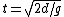[Latex: t=sqrt {2d/g}]

What is the velocity of an object that has traveled d meters? Equation: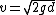[Latex: v=sqrt{2gd}]

What has been the average velocity of an object that has been falling for t seconds? Equation: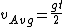[Latex: v_{Avg}=\frac{gt}{2}]

What has been the average velocity of an object that has fallen for distance d meters? Equation: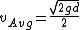[Latex: v_{Avg}=\frac{sqrt{2gd}}{2}]

Footnotes:

• It is assumed that the falling object in question has negligible mass.
• It is assumed that the object started freefall on the surface of the body (i.e., the initial distance from the body's center of gravity was the radius of the body)
• Mass and mean radius data from the NASA factsheet.